# Absolutely continuous measures

(diff) ← Older revision | Latest revision (diff) | Newer revision → (diff)

Suppose that on the measurable spacethere are given two measuresand(cf. also Measure). One says thatis absolutely continuous with respect to(denoted) if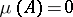implies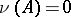for any set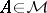. One also says thatdominates. If the measureis finite (i.e.), then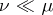if and only if for anythere exists asuch that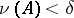whenever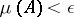.

The Radon–Nikodým theorem says that ifandare-finite measures and, then there exists a-integrable non-negative function(a density, cf. also Integrable function), called the Radon–Nikodým derivative, such that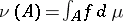. Two such densitiesand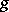may differ only on a null set (see Measure), i.e.. An example of a density (with respect to the Lebesgue measure on the interval, i.e. the length) is the function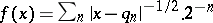, whereis the sequence of all rational numbers in this interval.

The measure is-finite ifis the union of a countable family of sets with finite measure.

Given a reference measureon, any measure may be decomposed into a sum of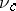and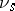with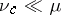and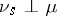, i.e. an absolutely continuous and a singular part. This is called the Lebesgue decomposition.

A set of non-zero measure that has no subsets of smaller, but still positive, measure is called an atom of the measure. It is a common mistake to claim that the singular part of a measure must be concentrated on points which are atoms. A singular measure may be atomless, as is shown by the measure concentrated on the standard Cantor set which puts zero on each gap of the set andon the intersection of the set with the interval of generation.

When some canonical measureonis fixed (as the Lebesgue measure on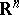or its subsets or, more generally, the Haar measure on a topological group), one says thatis absolutely continuous on, meaning that.

Two measures which are mutually absolutely continuous are called equivalent.